8 篇文章 1 订阅

# 0 前言

Go 是一门非常不错的语言，但是为了锻炼一下自己，使用 C++ 实现了书中的想法，项目地址：https://github.com/ivanallen/autumn

C++ 是一门非常灵活的语言，正好趁这个小项目，我可以天马行空的实现我的各种想法，应用到工程里。

# 1 特性

• 基于 C++ 17 语法实现。如果可能的话，我希望我能用上 C++ 20. 仅管目前用到的新特性不是很多，但我希望有些地方，能使用新特性来实现。或许你对新特性更加熟悉。
• 平台：当然是 Linux 和 Mac 都支持啦！（为什么不支持 Windows ? 求大神提 PR 改造！）
• 完善的注释。抱歉，我撒谎了，后面我一定会补上！
• 完善的 Debug 能力。是的，编写 Pratt Parsing 算法是个挑战，理解它如何工作也是一个挑战。你不可能通过 gdb 一行一行去调试。Autumn 项目希望能在 Debug 能力上帮助你理解 Pratt Parsing. 下面是一张截图。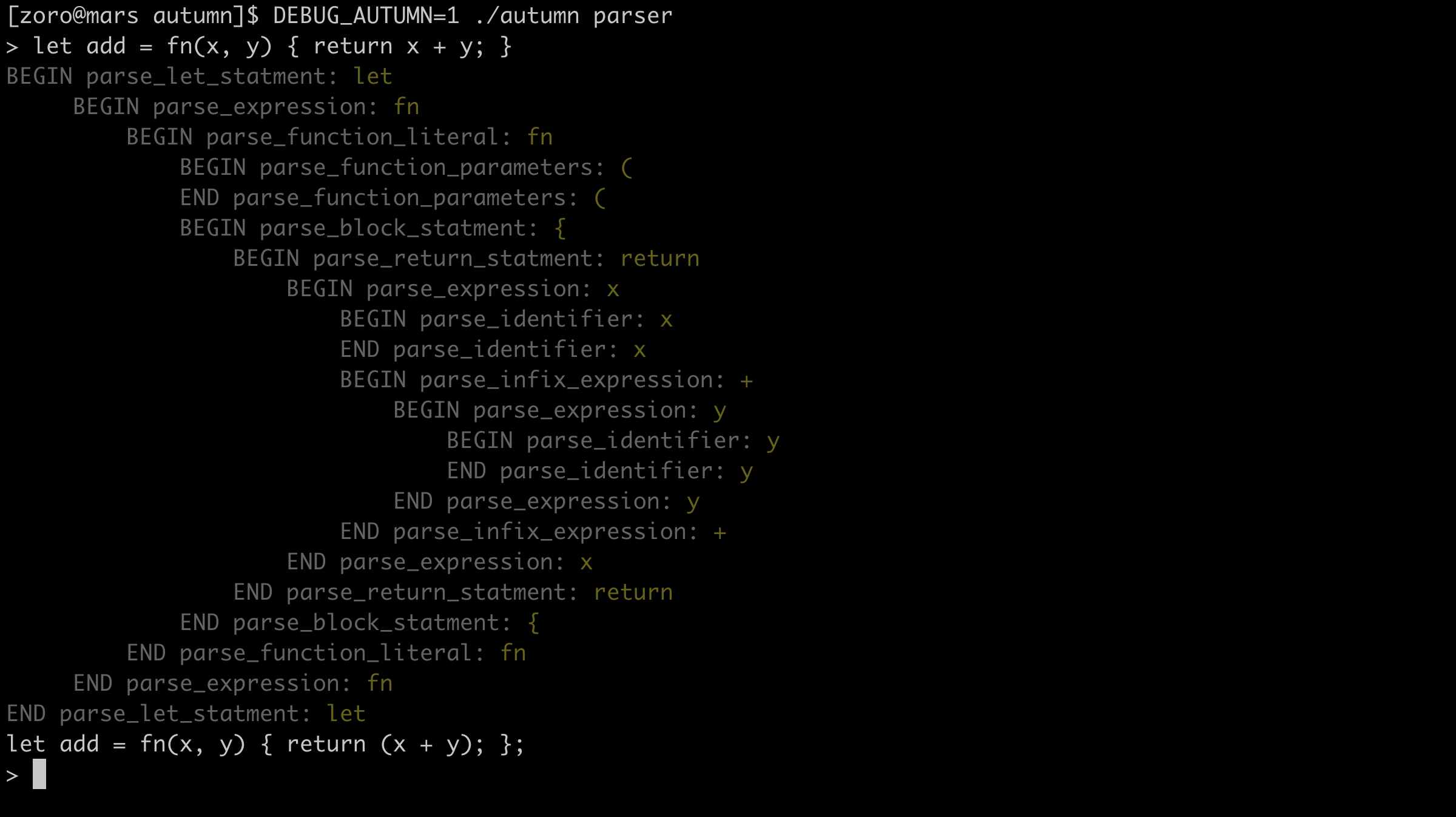• 高阶函数的支持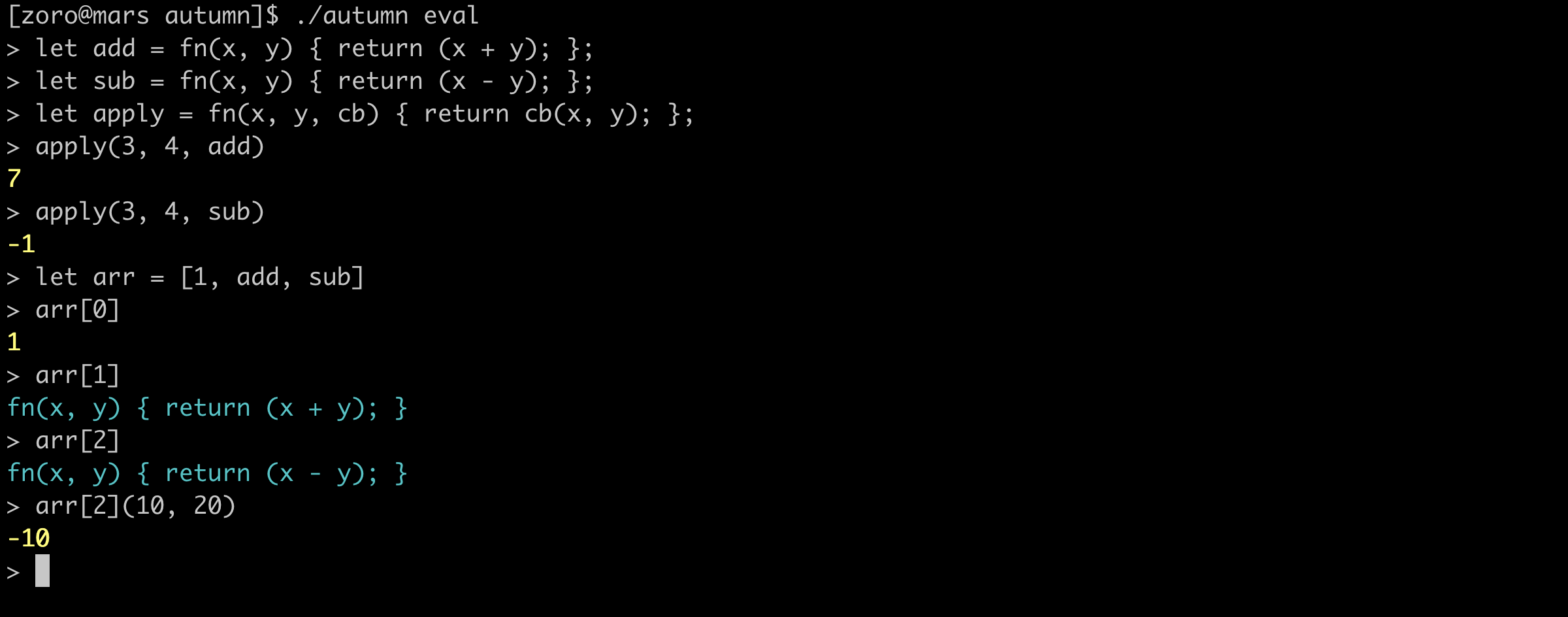• 闭包支持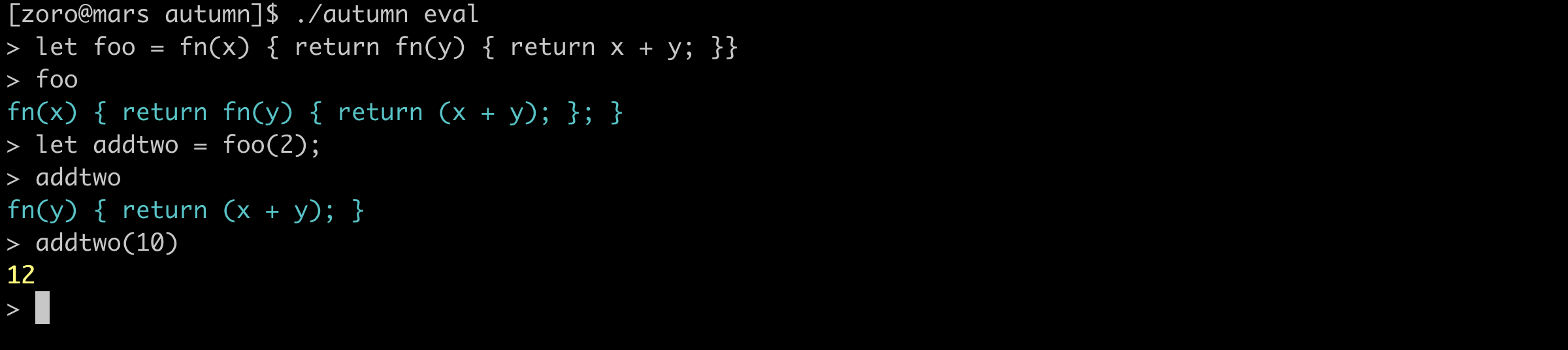# 2 快速开发

• 第一步当然是 fork & clone 这个项目到你的 Mac 或者 Linux 系统的主机上。
• GCC8 安装

# CentOS
$yum install readline-devel.x86_64$ yum install -y devtoolset-8-toolchain
$scl enable devtoolset-8 bash # Ubuntu$ sudo add-apt-repository -y ppa:ubuntu-toolchain-r/test
$sudo apt-get update -qq$ sudo apt-get install -qq g++-8
$apt-get install lib64readline-dev$ export CXX="g++-8"
$export CC="gcc-8"  • 编译 $ make

• 进入解释器
\$ ./autumn eval


# 3 一些酷炫的例子

## 3.1 map

let map = fn(arr, f) {
let iter = fn(arr, accumulated) {
if (len(arr) == 0) {
accumulated
} else {
iter(rest(arr), push(accumulated, f(first(arr))));
}
};
iter(arr, []);
};

// 压缩成一行的
let map = fn(arr, f) { let iter = fn(arr, accumulated) { if (len(arr) == 0) { accumulated } else { iter(rest(arr), push(accumulated, f(first(arr)))); }}; iter(arr, []);};

• 数组元素 double
let a = [1,2,3,4]
let double = fn(x) { x * 2 };
map(a, double)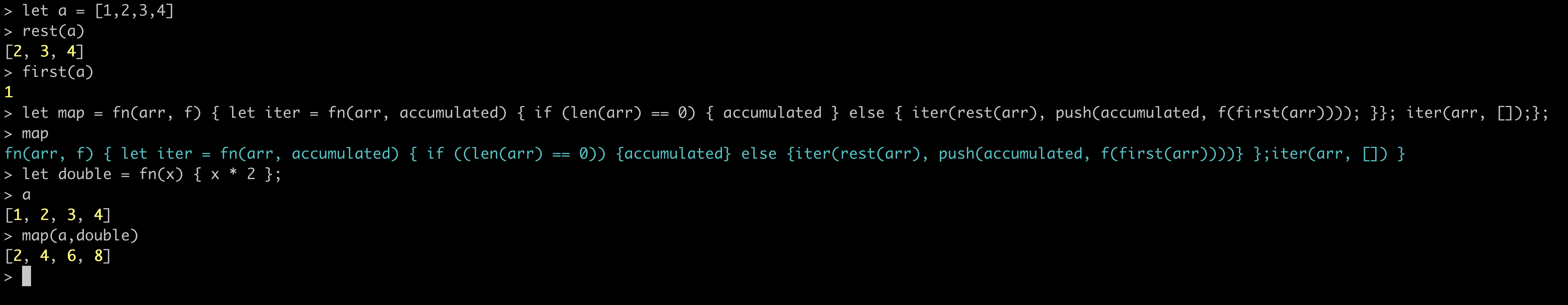## 3.2 filter

let filter = fn(arr, f) {
let iter = fn(arr, accumulated) {
if (len(arr) == 0) {
accumulated
} else {
let e = if (f(first(arr))) {
push(accumulated, first(arr));
} else {
accumulated
}
iter(rest(arr), e);
}
};
iter(arr, []);
};

// 压缩成一行的
let filter = fn(arr, f) { let iter = fn(arr, accumulated) { if (len(arr) == 0) { accumulated } else { let e = if (f(first(arr))) { push(accumulated, first(arr)); } else { accumulated } iter(rest(arr), e); }}; iter(arr, []);};

• 找到小于 4 的元素
let a = [1,5,3,6,3,8]
let lessThanFour = fn(x) { x < 4 }
filter(a, lessThanFour)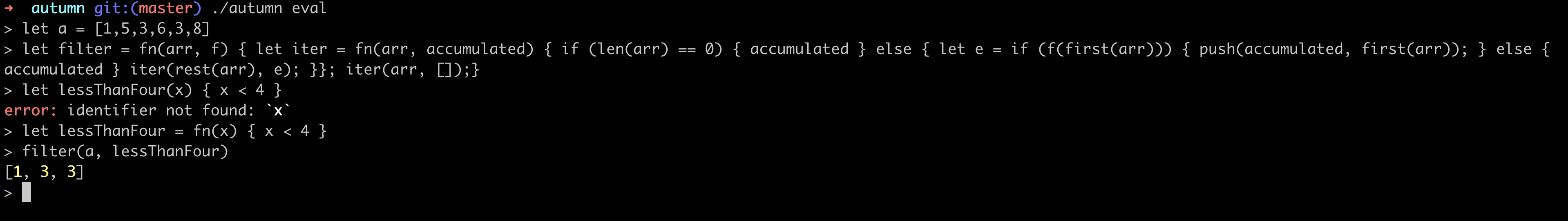• 快速排序
let quickSort = fn(arr) {
if (len(arr) == 0) {
return arr;
} else {
let smaller = filter(rest(arr), fn(x) { x <= head });
let bigger = filter(rest(arr), fn(x) { x > head });
return quickSort(smaller) + [head] + quickSort(bigger);
}
}

// 一行版本
let quickSort = fn(arr) {if (len(arr) == 0) {return arr;} else {let head = first(arr);let smaller = filter(rest(arr), fn(x) { x <= head });let bigger = filter(rest(arr), fn(x) { x > head });return quickSort(smaller) + [head] + quickSort(bigger);}}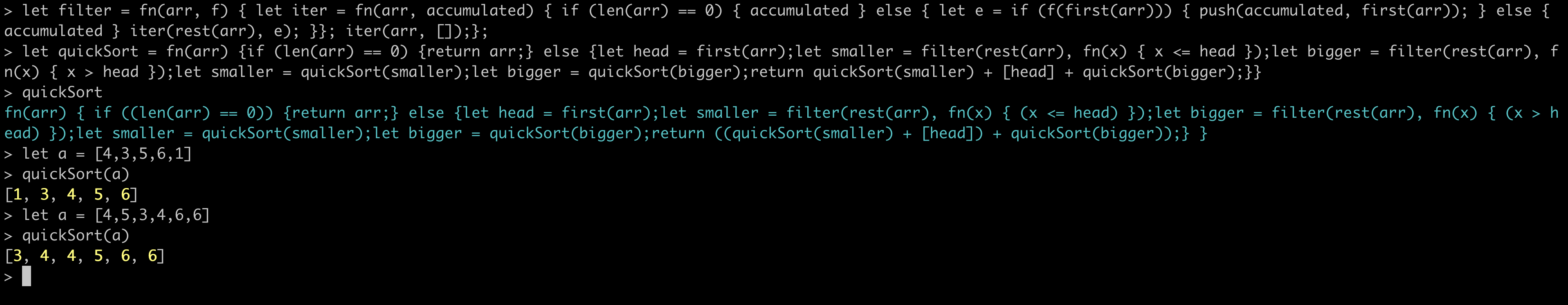# 4 尚未被支持的

• if … else if 结构（目前只支持了 if … else）
• 更多的内建函数 （目前实现了 len 函数等）
• 能像 Python 那样从文件读取代码执行
• ……

# 5 Q&A

• Q: 为什么不支持循环呢？
• A: autumn 被定位成函数式编程语言，所以不支持循环，不支持变量。
04-19
05-221万+01-02
09-122567
12-064168
10-27834
03-3181
10-134561
04-144728
10-182万+
05-27
01-05
05-159984
02-21159
07-0697
04-16621点击重新获取扫码支付余额充值